Quantum Computing

... from transistors to quantum supremacy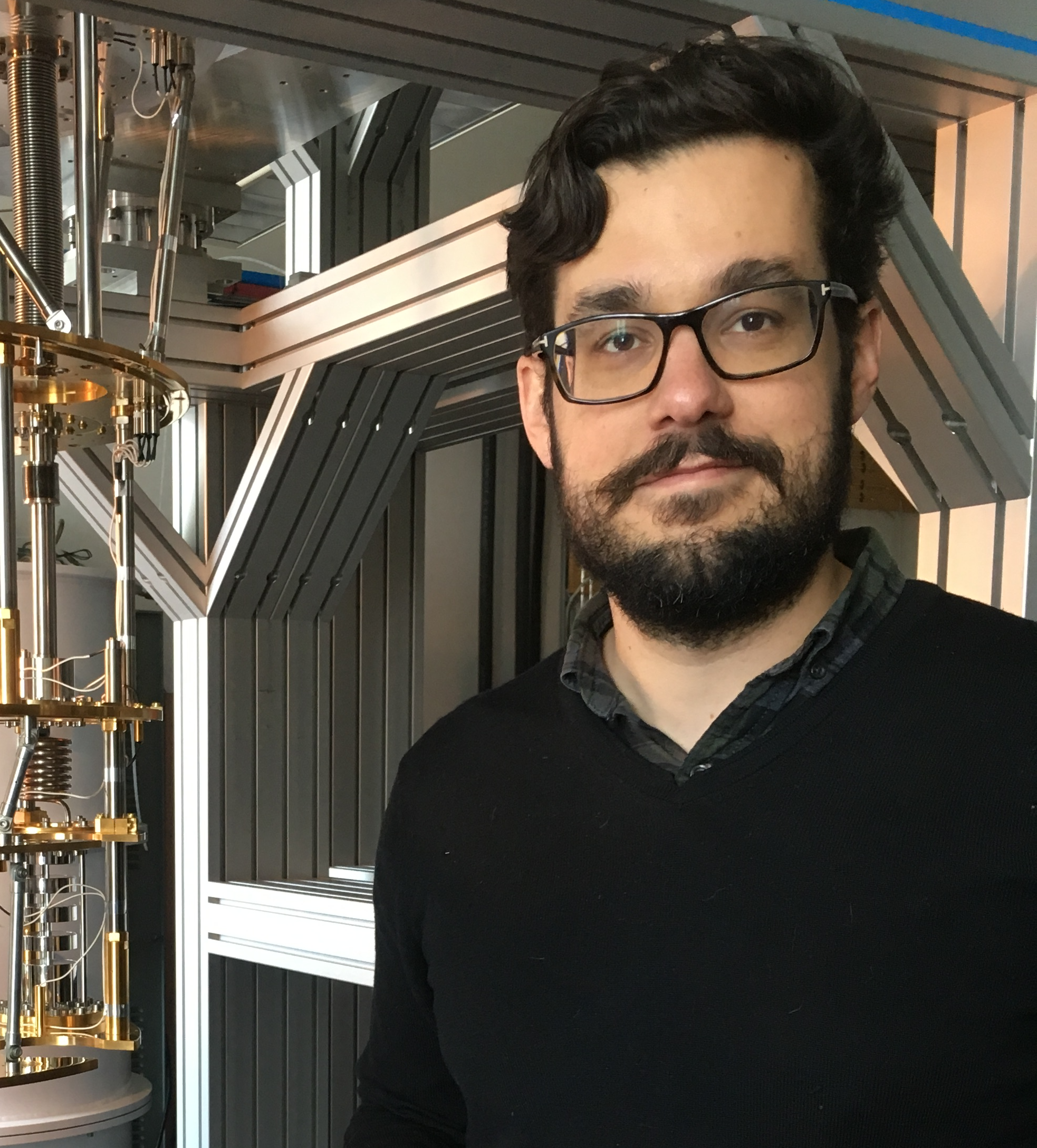Slides by Nik Hartman. Originally for Nerd Nite YVR #37.

Quantum supremacy

• When a quantum computer can accomplish something a classical computer cannot.
• Likely to show up in your newsfeed in the next 1-5 years.

First -- Let's talk classical computers

All the computer hardware we know and love today is based on the semiconductor transistor .

Transistors = Semiconductor JunctionsNow we can do calculations with binary numbers!

Before we do calculations... let's learn to count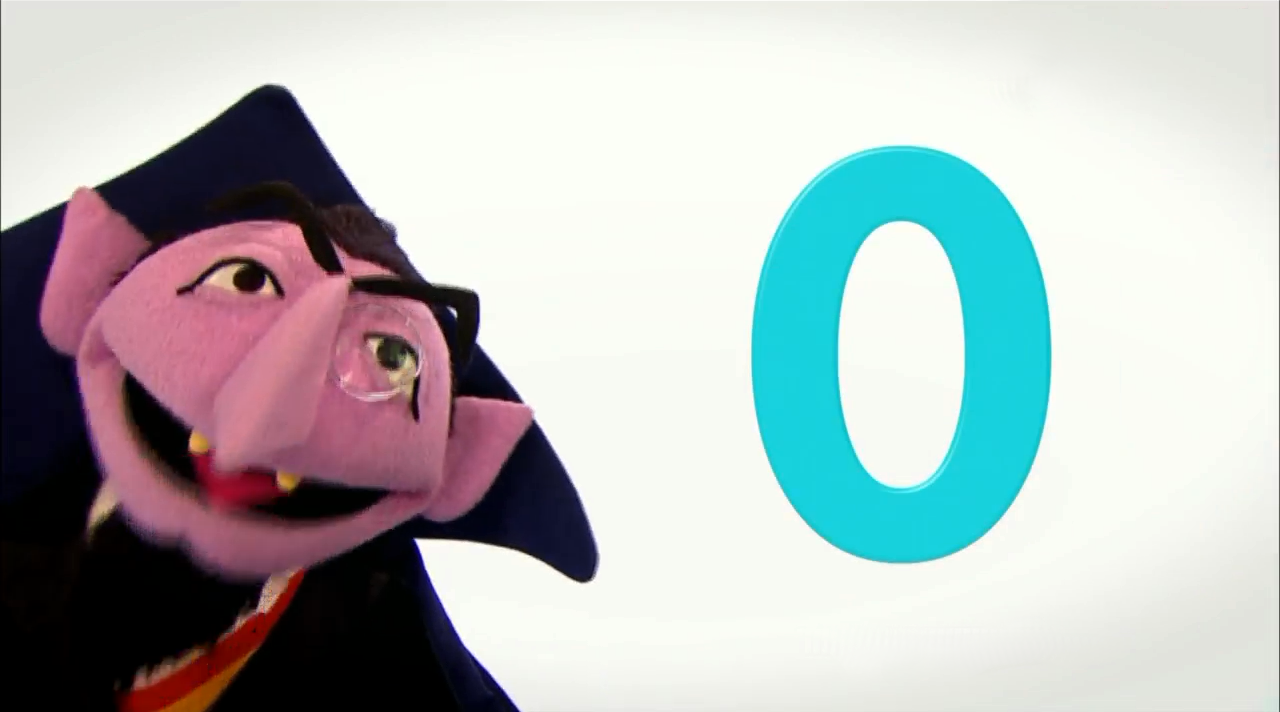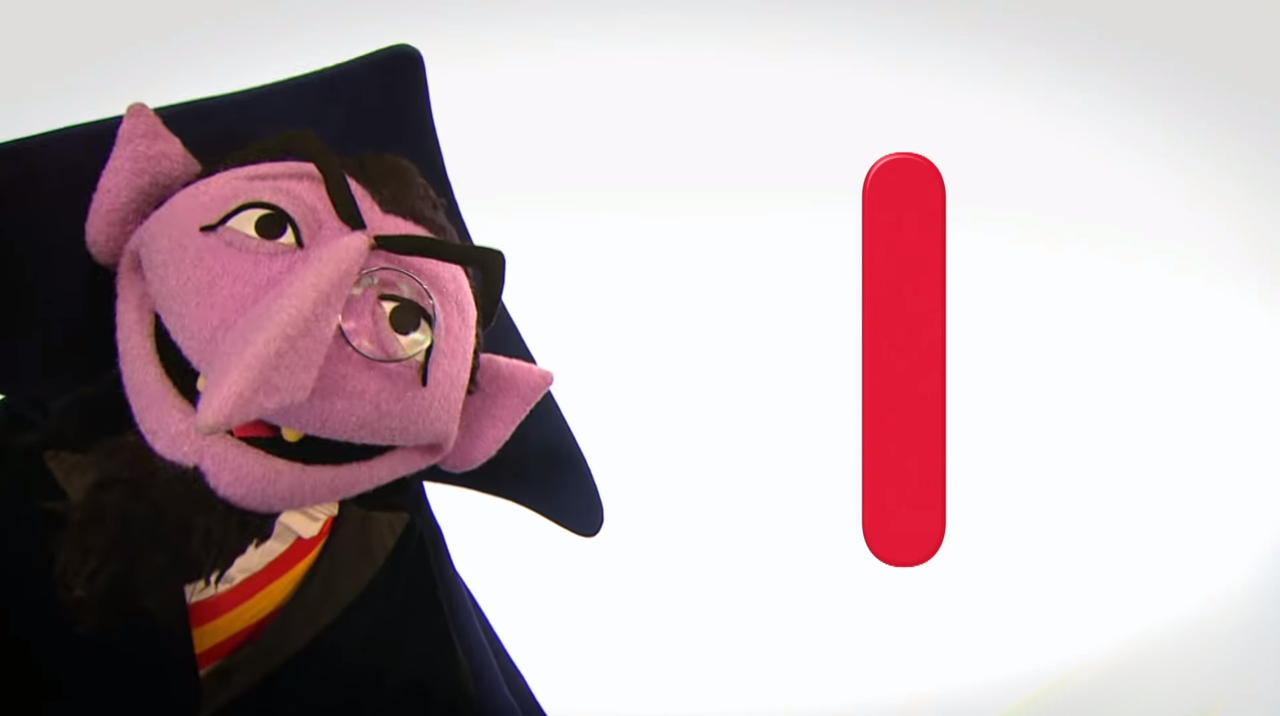$2^2=4$ $2^1=2$ $2^0=1$ SUM 0 0 0 0 0 0 1 1 0 1 0 2 0 1 1 3 1 0 0 4 1 0 1 5 1 1 0 6 1 1 1 7

Transistors to logic gates (if-then statements)AND-gate
A B OUTPUT
0 0 0
1 0 0
0 1 0
1 1 1

many possible logic gates can be created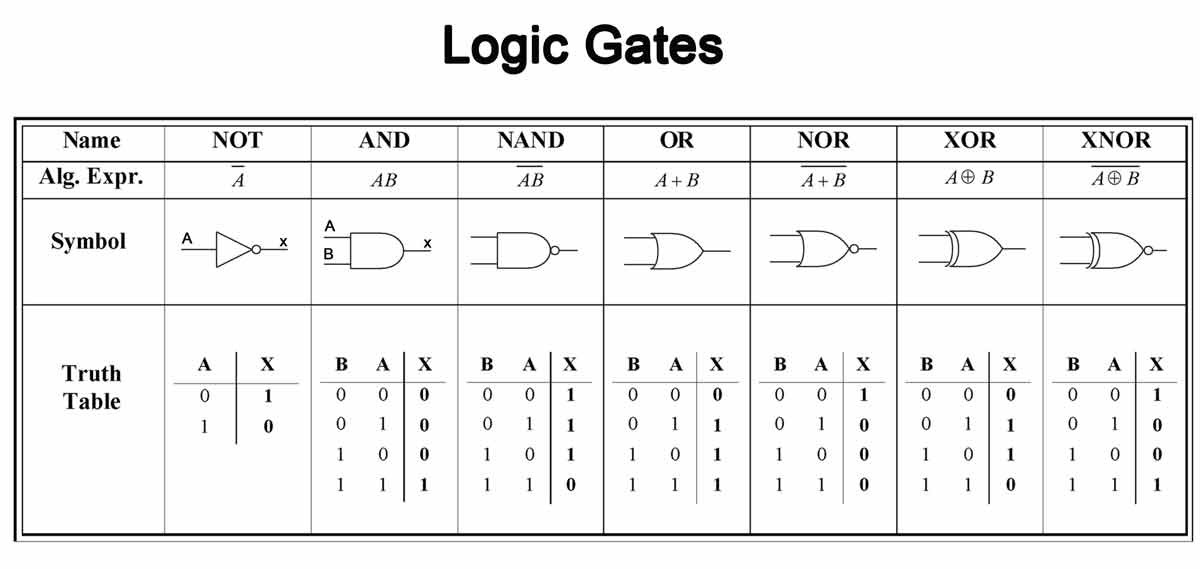EXAMPLE: 2 + 3 = ??? (or 010 + 011 = ???)2 + 3 = 5

but computers do more than add numbers...

• Many of the hardest computational problems are simulations of nature.
• Often we can be simplify and accept approximate results
• What about exact simulations of nature?
• To describe nature exactly we need quantum mechanics.

example: interesting (but difficult) simulation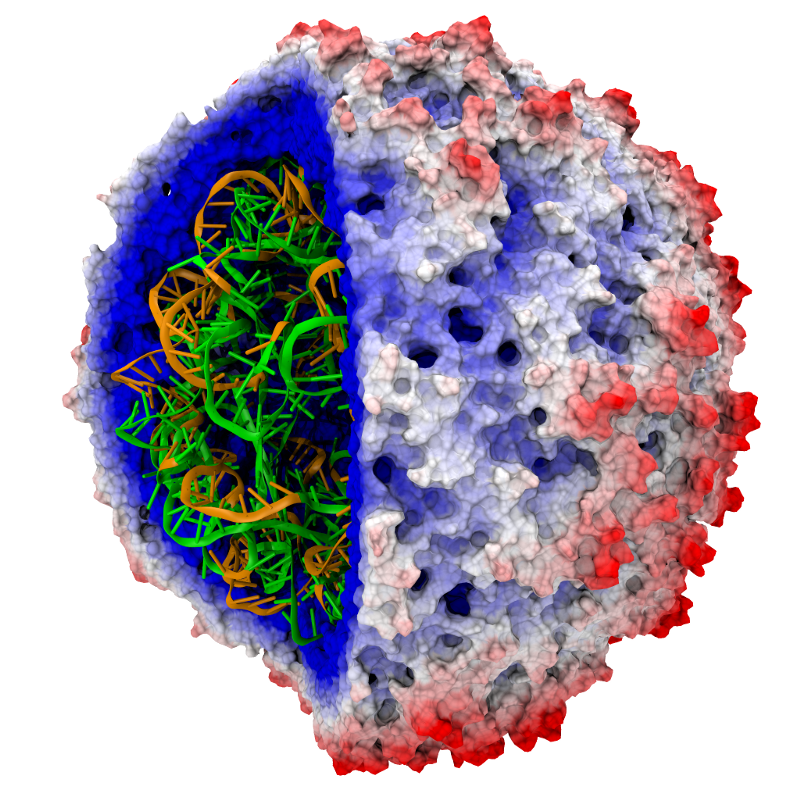• Simulating molecules for better/safer drugs
• Quanum mechanics determines molecule shape, interactions, and efficacy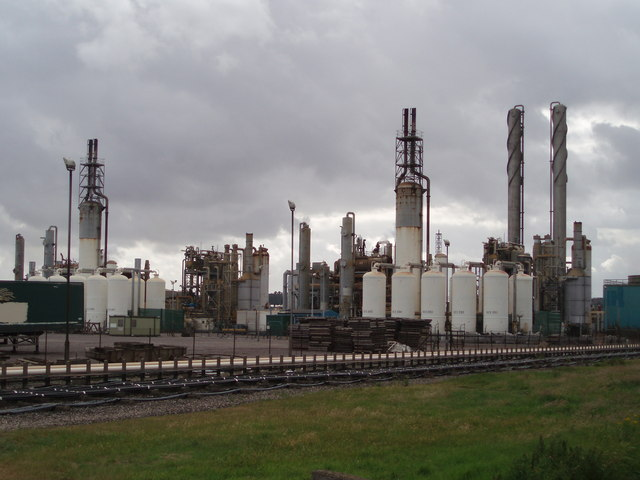Ammonia molecules and nitrogen fixation processes: $N_2$ --> $NH_3$. Why are plants so much better at this?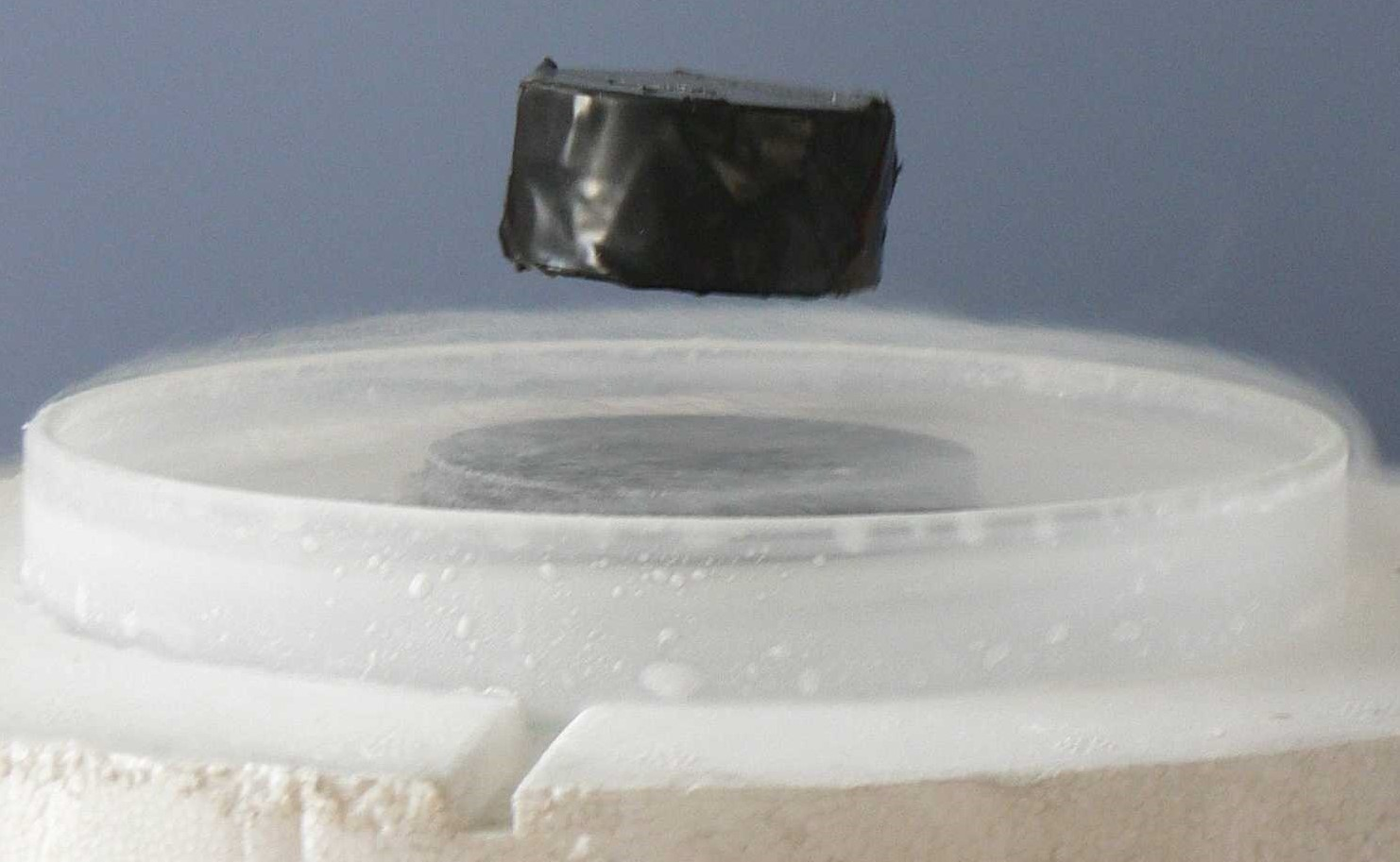Search for new materials with specific properties Room temperature superconductivity and other (less lofty) goals

Superposition

A fundamental property of the very small world.• The only results we can measure are $\uparrow$ or $\downarrow$
• Before measurement it can exist in a combination (superposition) of both states.

superposition = $a \cdot \downarrow + b \cdot \uparrow$

• $a$ and $b$ are the probabilities of measuring the atom pointing down or up

Superposition -- An Analogy*

superposition = $\frac{1}{2} \cdot LAMB + \frac{1}{2} \cdot LOBSTER$

• What happens when the waiter asks (measures) what you would like?
• Your uncertain order (superposition) collapses into a single decision.
• If this scenario could be recreated over and over -- 50% of the time you choose lamb, 50% lobster

* without any dead cats

more is different -- and may be impossible• A system of N atoms has $2^N$ possible configurations
• $2 \cdot 2 \cdot 2 \ldots 2 \cdot 2 \cdot 2 = 2^N$
• Any simulation must keep track of all of these configurations to determine most probable measurement result
• Adding another atom doubles the processing power needed

ok.. let's double the processing power!One limit on this plan

• 1 billion operations per second => smartphone
• Uses about as much power as an LED light bulb
• Today's supercomputers: 100 million-billion ($10^{17}$) operations per second
• About as much power as New York City
• $2.5 million CAD in electricity per year • That is roughly 1 million billion ($10^{15}$) Joules per year • World produces about 100 billion billion ($10^{21}$) Joules per year transistor size limits • Modern CPU has 10 billion ($10^{10}$) transistors. • Supercomputers use 100,000 processors in parallel. • About$10^{15}$transistors in total. • Number of cells in 10 people.• Probability of electron(s) spontaneously hopping across$\sim e^{-kL}$• L = channel length • k = electron energy What do we get for all that power?Simulate interactions between 45 atoms Using current technology and all of the world's electricity, we can get to about 70 atoms. Richard Feynman suggests a way out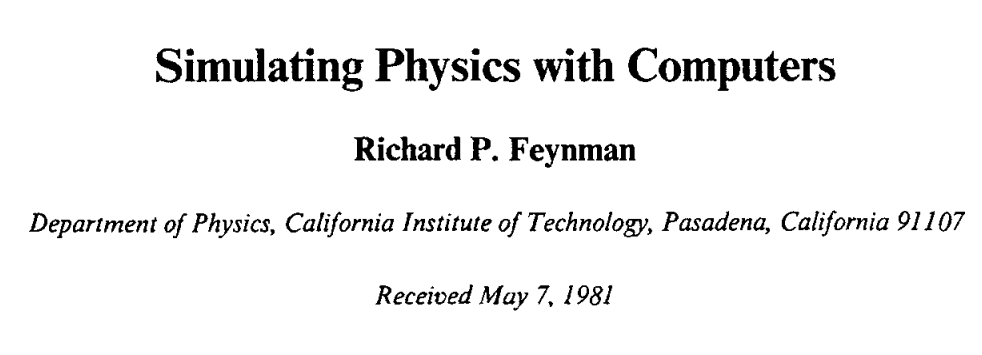If simulating quantum mechanics on a classical computer is too costly, build a quantum computer. Transistors + Quantum Mechanics = quantum bits• Always 0 or 1 • Channel open or closed • Any superposition of 0 and 1 • Atom up or down A quantum processor needs more than one qubit • Qubits can be combine to make more complicated superpositions • Single atom superposition: $$superposition = a_0 \cdot \downarrow + a_1 \cdot \uparrow$$ • Many atoms forming a single superposition are entangled. • Multiple (3) atom entangled superposition: $$superposition = a_0 \cdot \downarrow\downarrow\downarrow + a_1 \cdot \downarrow\downarrow\uparrow + a_2 \cdot \downarrow\uparrow\downarrow + a_3 \cdot \uparrow\downarrow\downarrow + \\ a_4 \cdot \downarrow\uparrow\uparrow + a_5 \cdot \uparrow\downarrow\uparrow + a_6 \cdot \uparrow\uparrow\downarrow + a_7 \cdot \uparrow\uparrow\uparrow$$ • We can perform logical gates (if-then statements) on these quantum bits! • Connect quantum bits with a gates (if-then statements, e.g. AND, NAND, XOR, ...) • We invented a quantum computer! A sensibly sized simulation of nature • Entangled state of N-qubits is a superposition of all$2^N\$ configurations.
• $$superposition = a_0 \cdot \downarrow\downarrow\downarrow + a_1 \cdot \downarrow\downarrow\uparrow + a_2 \cdot \downarrow\uparrow\downarrow + a_3 \cdot \uparrow\downarrow\downarrow + \\ a_4 \cdot \downarrow\uparrow\uparrow + a_5 \cdot \uparrow\downarrow\uparrow + a_6 \cdot \uparrow\uparrow\downarrow + a_7 \cdot \uparrow\uparrow\uparrow$$
• Quantum computer needs only N qubits to simulate N quantum mechanical objects.
• About 50 qubits can do something impossible for a classical computer!

Real Qubit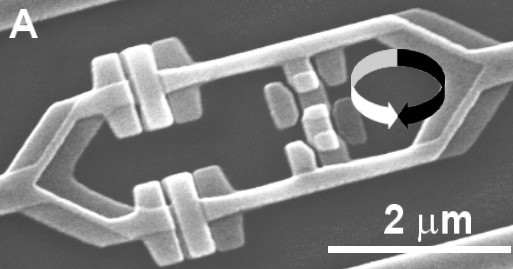• Superconducting Ring
• CW current = 0
• CCW current = 1
• Built on pieces of semiconductor
• Easy to connect many qubits
• Just like connecting many transistors in our classical computer

Real QubitsTrapped Atom: stable, difficult to coupleSuperconducting Ring: not stable, easy to couple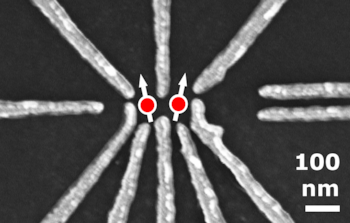Electron Spins: kind of stable, kind of hard to couple

Real Quantum Computers

• Intel -- 49 qubits
• IBM -- 50 qubits
• Google -- 72 qubits
• Not quite enough... yet
• Superconducting states stable for around 100 micro-seconds
• Must stay very cold and isolated from environment
• Correcting errors requires additional qubits
• Need 100-500 for 50 atom simulation

What about our hometown heros?!

D-Wave makes quantum annealing processors. Not universal quantum comptuers.• Define interactions between qubits.
• Example: spins have lower energy when pointed in the same direction as neighbor(s).